Courses

# RD Sharma Solutions: Exercise 1.3- Knowing Our Numbers Class 6 Notes | EduRev

## Class 6 : RD Sharma Solutions: Exercise 1.3- Knowing Our Numbers Class 6 Notes | EduRev

The document RD Sharma Solutions: Exercise 1.3- Knowing Our Numbers Class 6 Notes | EduRev is a part of the Class 6 Course Mathematics (Maths) Class 6.
All you need of Class 6 at this link: Class 6

Q.1. Estimate each of the following using general rule:

(a) 730 + 998

(b) 796 − 314

(c) 12, 904 + 2, 888

(d) 28, 292 − 21, 496

Make ten more such examples of addition, subtraction and estimation of their outcome.

Ans:

(a) 730 + 998

By rounding off to hundreds, 730 rounds off to 700 and 998 rounds off to 1000.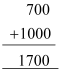(b) 796 − 314

By rounding off to hundreds, 796 rounds off to 800 and 314 rounds off to 300.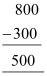(c) 12904 + 2822

By rounding off to thousands, 12904 rounds off to 13000 and 2822 rounds off to 3000.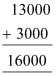(d) 28,296 − 21,496

By rounding off to nearest thousands, 28296 rounds off to 28000 and 21496 rounds off to 21000.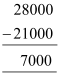Q.2. Give a rough estimate (by rounding off to nearest hundreds) and also a closer estimate (by rounding off to nearest tens):

(a) 439 + 334 + 4, 317

(b) 1,08, 734 − 47, 599

(c) 8325 − 491

(d) 4, 89, 348 − 48, 365

Make four more such examples.

Ans:

(a) 439 + 334 + 4317

Rounding off to nearest hundreds, 439, 334, and 4317 may be rounded off to 400, 300, and 4300 respectively.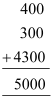Rounding off to nearest tens, 439, 334, and 4317 may be rounded off to 440, 330, and 4320 respectively.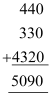(b) 1,08,734 − 47,599

Rounding off to hundreds, 1,08,734 and 47,599 may be rounded off to 1,08,700 and 47,600 respectively.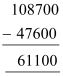Rounding off to tens, 1,08,734 and 47,599 may be rounded off to 1,08,730 and 47,600 respectively.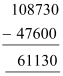(c) 8325 − 491

Rounding off to hundreds, 8325 and 491 may be rounded off to 8300 and 500 respectively.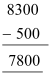Rounding off to tens, 8325 and 491 may be rounded off to 8330 and 490 respectively.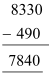(d) 4,89,348 − 48,365

Rounding off to hundreds, 489348 and 48365 may be rounded off to 489300 and 48400 respectively.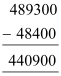Rounding off to tens, 489348 and 48365 may be rounded off to 489350 and 48370 respectively.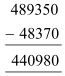Q.3. Estimate the following products using general rule:

(a) 578 × 161

(b) 5281 × 3491

(c) 1291 × 592

(d) 9250 × 29

Ans:

(a) 578 × 161

Rounding off by general rule, 598 and 161 may be rounded off to 600 and 200 respectively.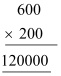(b) 5281 × 3491

Rounding off by general rule, 5281 and 3491 may be rounded off to 5000 and 3000 respectively.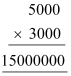(c) 1291 × 592

Rounding off by general rule, 1291 and 592 may be rounded off to 1000 and 600 respectively.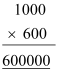(d) 9250 × 29

Rounding off by general rule, 9250 and 29 may be rounded off to 9000 and 30 respectively.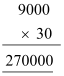Offer running on EduRev: Apply code STAYHOME200 to get INR 200 off on our premium plan EduRev Infinity!

## Mathematics (Maths) Class 6

191 videos|224 docs|43 tests

,

,

,

,

,

,

,

,

,

,

,

,

,

,

,

,

,

,

,

,

,

;